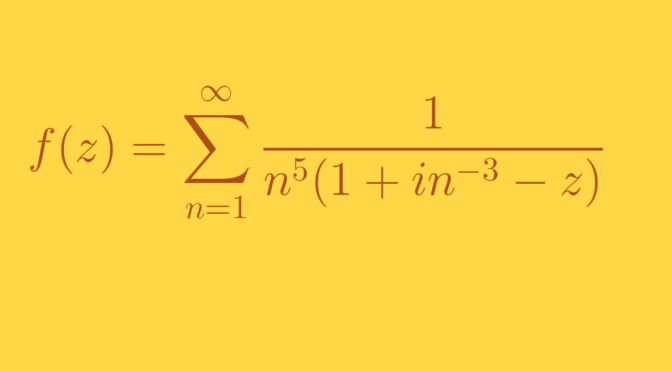# A power series converging everywhere on its circle of convergence defining a non-continuous function

Consider a complex power series $$\displaystyle \sum_{k=0}^\infty a_k z^k$$ with radius of convergence $$0 \lt R \lt \infty$$ and suppose that for every $$w$$ with $$\vert w \vert = R$$, $$\displaystyle \sum_{k=0}^\infty a_k w^k$$ converges.

We provide an example where the power expansion at the origin $\displaystyle f(z) = \sum_{k=0}^\infty a_k z^k$ is discontinuous on the closed disk $$\vert z \vert \le R$$.

The function $$f$$ is constructed as an infinite sum $\displaystyle f(z) = \sum_{n=1}^\infty f_n(z)$ with $$f_n(z) = \frac{\delta_n}{a_n-z}$$ where $$(\delta_n)_{n \in \mathbb N}$$ is a sequence of positive real numbers and $$(a_n)$$ a sequence of complex numbers of modulus larger than one and converging to one. Let $$f_n^{(r)}(z)$$ denote the sum of the first $$r$$ terms in the power series expansion of $$f_n(z)$$ and $$\displaystyle f^{(r)}(z) \equiv \sum_{n=1}^\infty f_n^{(r)}(z)$$.

We’ll prove that:

1. If $$\sum_n \delta_n \lt \infty$$ then $$\sum_{n=1}^\infty f_n^{(r)}(z)$$ converges and $$f(z) = \lim\limits_{r \to \infty} \sum_{n=1}^\infty f_n^{(r)}(z)$$ for $$\vert z \vert \le 1$$ and $$z \neq 1$$.
2. If $$a_n=1+i \epsilon_n$$ and $$\sum_n \delta_n/\epsilon_n < \infty$$ then $$\sum_{n=1}^\infty f_n^{(r)}(1)$$ converges and $$f(1) = \lim\limits_{r \to \infty} \sum_{n=1}^\infty f_n^{(r)}(1)$$
3. If $$\delta_n/\epsilon_n^2 \to \infty$$ then $$f(z)$$ is unbounded on the disk $$\vert z \vert \le 1$$.

First, let’s recall this corollary of Lebesgue’s dominated convergence theorem:

Let $$(u_{n,i})_{(n,i) \in \mathbb N \times \mathbb N}$$ be a double sequence of complex numbers. Suppose that $$u_{n,i} \to v_i$$ for all $$i$$ as $$n \to \infty$$, and that $$\vert u_{n,i} \vert \le w_i$$ for all $$n$$ with $$\sum_i w_i < \infty$$. Then for all $$n$$ the series $$\sum_i u_{n,i}$$ is absolutely convergent and $$\lim_n \sum_i u_{n,i} = \sum_i v_i$$.

### Proof of result (1)

Let’s fix $$\vert z \vert \le 1$$ with $$z \neq 1$$. The sum of the first $$r$$ terms in the power series expansion of $$f_n(z)$$ about the origin is equal to $f_n^{(r)}(z) = \delta_n \frac{1-\left( z/a_n \right)^r}{a_n-z}.$ As $$\vert a_n \vert > 1$$ we have $\left\vert f_n^{(r)}(z) \right\vert = \left\vert \delta_n \frac{1-\left( z/a_n \right)^r}{a_n-z} \right\vert \le \frac{2 \delta_n}{\vert a_n – z \vert}$

And as $$\lim_n a_n = 1$$, the right hand side of above inequality is bounded by a multiple of $$\delta_n$$. With the hypothesis $$\sum_n \delta_n < \infty$$ that proves that the series $$\sum_n f_n^{(r)}(z)$$ is absolutely convergent for all $$r \ge 0$$. Also for all $$n \in \mathbb N$$ we have $\lim\limits_{r \to \infty} f_n^{(r)}(z) = \lim\limits_{r \to \infty} \delta_n \frac{1-\left( z/a_n \right)^r}{a_n-z} = \frac{\delta_n}{a_n-z}.$ Using the corollary of the dominated converge theorem mentioned above we get $f(z) = \sum_{n=1}^\infty \frac{\delta_n}{a_n-z} = \lim\limits_{r \to \infty} \sum_{n=1}^\infty f_n^{(r)}(z)$ proving that $$f(z)$$ is a power series on the open disk $$\vert z \vert <1$$ converging on its circle of convergence for all $$z \neq 1$$.

### Proof of result (2)

Let’s now consider the case $$z = 1$$. We have $\left\vert f_n^{(r)}(1) \right\vert = \left\vert \delta_n \frac{1-\left( 1/a_n \right)^r}{a_n-1} \right\vert \le \frac{2 \delta_n}{\epsilon_n}$ as $$\vert a_n – 1 \vert = \epsilon_n$$ for all $$n \in \mathbb N$$. With the hypothesis $$\sum_n \delta_n/\epsilon_n < \infty$$, we get that $$\sum_n f_n^{(r)}(1)$$ is absolutely convergent for all $$r \ge 0$$. Applying again dominated convergence theorem, we find that that the power series of $$f(z)$$ about the origin converges for $$z=1$$.

### Proof of result (3)

Finally we have to prove that $$f(z)$$ is unbounded on the disk $$\vert z \vert \le 1$$ if $$\delta_n/\epsilon_n^2 \to \infty$$.

For $$m \in \mathbb N$$ the real part of $$\frac{1}{a_m – z}$$ is $$\frac{1- \Re z}{\vert a_m – z \vert^2}$$, hence is non negative for $$\vert z \vert \le 1$$. While for $$q_n=a_n/ \left\vert a_n \right\vert$$: $\Re f(q_n) \ge \Re f_n(q_n)=\frac{\delta_n}{\sqrt{1+\epsilon_n^2}(\sqrt{1+\epsilon_n^2}-1)}\ge\frac{2\delta_n}{\epsilon_n^2\sqrt{1+\epsilon_n^2}}.$ If $$\delta_n/\epsilon_n^2 \to \infty$$ we conclude that $$\Re f(q_n)$$ diverges to $$\infty$$.

### A precise example

An example of function satisfying the three conditions mentioned above is $f(z)=\sum_{n=1}^\infty\frac1{n^5(1+in^{-3}-z)}$ for which

• $$\delta_n = \frac{1}{n^5}$$
• $$a_n = 1 + i n^{-3}$$